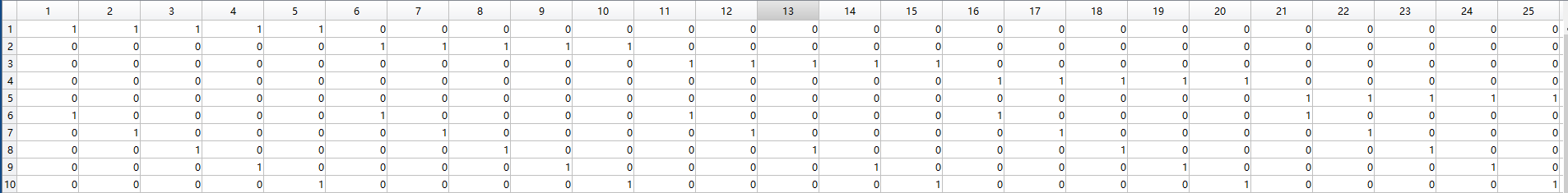• 关于MATLAB随机函数：MATLAB含有一些生成随机数的函数：一、rand ():生成(0,1)区间上均匀分布的随机变量rand(5,1) %生成随机数排列的列向量。rand(5)%生成5行5列的随机矩阵。rand(5,2)%生成5行2列的随机矩阵。x=...
关于MATLAB的随机函数：MATLAB含有一些生成随机数的函数：一、rand ():生成(0,1)区间上均匀分布的随机变量rand(5,1) %生成随机数排列的列向量。rand(5)%生成5行5列的随机矩阵。rand(5,2)%生成5行2列的随机矩阵。x=rand(10000,1);hist(x);%绘图，生成随机数的分布二、randn ():生成(0,1)区间上正态分布的随机变量randn(5,1)%生成随机数排列的列向量randn(5)%生成5行5列的随机矩阵。randn(5,2)%生成5行2列的随机矩阵。x=randn(10000,1);hist(x);%绘图，生成随机数的分布三、连续型分布随机函数unifrnd ():生成某个区间上均匀分布的随机变量unifrnd(-2,3,5,1)%在(-2,3)区间内生成一列随机变量unifrnd(-2,3,5)%在(-2,3)区间内生成5行5列的随机变量unifrnd(-2,3,5,2)%在(-2,3)区间内生成5行2列的随机变量x=unifrnd(-2,3,100000,1);hist(x,50)%绘图，生成随机数的分布normrnd ():生成指定均值，标准差的正态分布的随机变量normrnd(2,3,5,1)%生成指定均值为2，标准差为3的正态分布的5行1列随机变量normrnd(2,3,5)%生成指定均值为2，标准差为3的正态分布的5行5列的随机变量normrnd(2,3,5,2)%生成指定均值为2，标准差为3的正态分布的5行2列的随机变量x=unifrnd(-2,3,100000,1);hist(x,50)%绘图，生成随机数的分布chi2rnd():生成的随机数服从自由度为v的卡方分布chi2rnd(5,5,1)%生成服从自由度为5的卡方分布的5行1列随机变量chi2rnd(5,5)%生成服从自由度为5的卡方分布的的5行5列的随机变量chi2rnd(5,5,2)%生成服从自由度为5的卡方分布的5行2列的随机变量x=chi2rnd(5,100000,1);hist(x,50)%绘图，生成随机数的分布frnd():生成的随机数服从参数为w,v的t分布frnd(3,5,5,1)%生成服从参数为(3,5)的f分布的5行1列随机变量frnd(3,5,5)%生成服从参数为(3,5)的f分布的的5行5列的随机变量frnd(3,5,5,2)%生成服从参数为(3,5)的f分布的5行2列的随机变量x=frnd(3,5,10000,1);hist(x,50)%绘图，生成随机数的分布trnd():生成的随机数服从参数为v的t分布trnd(5,5,1)%生成服从参数为5的t分布的5行1列随机变量trnd(5,5)%生成服从参数为5的t分布的的5行5列的随机变量trnd(5,5,2)%生成服从参数为5的t分布的5行2列的随机变量x=trnd(5,100000,1);hist(x,50)%绘图，生成随机数的分布同理：betarnd()%生成服从参数为(w,v)的Beta分布的随机数exprnd() %生成服从参数为mu的指数分布的随机数gamma()%生成服从参数为(w,v)的Gamma分布的随机数lognrd()%生成服从参数为(mu,sigma)的对数正态分布的随机数raylrnd()%生成服从参数为w的瑞利分布的随机数wblrnd()%生成服从参数为(scale,shape)的对数正态分布的随机数......四、离散型分布随机函数unidrnd()%生成服从离散均匀分布的随机数unidrnd(5,5,1) %生成一列随机变量unidrnd(5,5) %生成5行5列的随机变量unidrnd(5,5,2) %生成5行2列的随机变量x=unidrnd(9,100000,1);hist(x,9) %绘图，生成随机数的分布binornd() %生成的随机数服从参数为(N,p)的二项分布binornd(10,0.3,5,1)%生成5个随机数排列的列向量%注：上述语句生成的随机数所服从的参数为(10,0.3)的二项分布binornd(10,0.3,5) %生成5行5列的随机数矩阵%注：上述语句生成的随机数所服从的参数为(10,0.3)的二项分布binornd(10,0.3,[5,2])%生成一个5行2列的随机数矩阵%注：上述语句生成的随机数所服从的参数为(10,0.3)的二项分布x=binornd(10,0.45,100000,1);hist(x,11);%绘图，生成随机数的分布geornd()%此函数生成服从几何分布的随机数。geornd(0.4,5,1) %生成5个随机数排列的列向量%注：上述语句生成的随机数所服从的参数为(0.4)的二项分布geornd(0.4,5) %生成5行5列的随机数矩阵%注：上述语句生成的随机数所服从的参数为(0.4)的二项分布geornd(0.4,[5,2]) %生成一个5行2列的随机数矩阵%注：上述语句生成的随机数所服从的参数为(0.4)的二项分布x=geornd(0.4,100000,1);hist(x,50)%绘图，生成随机数的分布poissrnd() %生成服从泊松(Poisson)分布的随机数poissrnd(2,5,1) %生成5个随机数排列的列向量%注：上述语句生成的随机数所服从的参数为(2)的泊松分布poissrnd(2,5) %生成5行5列的随机数矩阵%注：上述语句生成的随机数所服从的参数为(2)的泊松分布poissrnd(5,[5,2])%生成一个5行4列的随机数矩阵%注：上述语句生成的随机数所服从的参数为(2)的泊松分布x=poissrnd(2,100000,1);hist(x,50);%绘图，生成随机数的分布展开全文• 问题描述：Matlab如何生成互不相同的随机整数我的问题是生成1到91之间的互不相同的个数从1到90的随机整数列,并且每个数不能等于771个回答分类：综合2014-12-15问题解答：我来补答有一点瑕疵,生成两位数的地方有点...
问题描述：Matlab如何生成互不相同的随机整数我的问题是生成1到91之间的互不相同的个数从1到90的随机整数列,并且每个数不能等于771个回答分类：综合2014-12-15问题解答：我来补答有一点瑕疵,生成两位数的地方有点错误,判断有无重复数的地方也有点错误,而且可以进行简化.修改了的地方我做了注释.(粘贴过来时出错了,我重新粘贴了下)#include#include#include#includeusing namespace std;void main(){int a,m;srand(time(0));int n;int i;for( n=0;n<10;n++){a[n]=rand()%90+10; //这样产生的数一定是个两位的整数for(i=0;iif(a[n]==a[i]) //如果有重复的数,则重新生成一个数,并重新判断有无重复的数}/*这段程序作废while(a[n]<10){a[n]=rand()%100;}for(n=0;n<10;n++){m=n+1;for(;m<10;m++){while(a[n]==a[m]){a[m]=rand()%100;}}} 这段程序作废*/cout<for(n=0;n<10;n++){cout<}cout<cout<for(n=0;n<10;n++){m=2;while(m<=(int)sqrt((double)a[n]) && a[n]%m!=0) //加上(double){m++;}if(m>(int)sqrt((double)a[n])) //加上(double)cout<
展开全文• 用法 r=myrand(a,b) a 和 b 是范围，例如，如果我们想生成 370 到 55780 之间的随机整数，我们调用函数： r=myrand(370,55780) 我们还可以根据需要限制列表。 例如，如果我们只想要 370 到 55780 之间的 10 个随机...
• MATLAB提供了多种生成随机整数的方法，下面我们就着重介绍几种常用的方法。 方法一：rand() rand()函数常用来生成0-1之间的随机矩阵，那么我们完全也可以用来生成我们想要的任意大小、任意个数的随机整数...

MATLAB提供了多种生成随机整数的方法，下面我们就着重介绍几种常用的方法。

方法一：rand()

rand()函数常用来生成0-1之间的随机矩阵，那么我们完全也可以用来生成我们想要的任意大小、任意个数的随机整数。

1，生成0-5之间的10个随机整数

在matlab主窗口中输入round(rand(1,10)*5) 回车2，生成1个随机整数，,

命令  round(rand(1,1)*5)  回车3，生成-5-4之间的10个随机整数

在matlab主窗口中输入   round(rand(1,10)*9)-5  回车我们可以看到生成的是在-5到4之间的随机整数！

方法二： randint()

randint()函数可以生成任意区间任意维数的随机整数矩阵，下面我们就举例验证之.

1,生成1个5-9之间的随机整数

在主窗口中输入 randint(1,1,[5 9])回车2，生成10个5到20之间的整数

在主窗口中输入  randint(1,10,[10 20])  回车黄色警告的意思是这个函数未来可能会被MATLAB移除，请用rand()函数，当然我们现在不熟悉rand()函数，还可以继续用这个函数。

3，生成10个-7到15之间的随机整数

在主窗口中输入  randint(1,10,[-7 15]) 回车结论：randint()函数其实是rand()函数的特殊版本，randint()能够生成的所有整数rand()函数都可以做到，不过需要我们自己去手动进行取整以及区间变换操作，randint()函数用起来方便，但是未来有可能这个函数被删除，所有希望大家也多去了解一下rand()函数。

展开全文• 程序快速输出 a 到 b 范围内的 n 个随机整数。 整数是从均匀分布中抽取的，以使选择整数的可能性相等。 该程序旨在处理非常大的整数范围并且仅选择这些整数中的非常少的数量时特别快。 用户指定输出是排序还是随机...
• 该包包含以下geenerators（在p9v2之前只有randorg）： (1) RANDORG - 真正的随机整数(2) SEQORG - 随机定位的整数序列(3) STRORG - 随机字符串 有关详细信息，请参阅每个功能的帮助有关示例，请参阅附加图像（仅限 ...
• 返回从指定集合中均匀绘制的随机元素矩阵。 可以在更换或不更换的情况下进行采样。 （此功能旨在填补 RANDINT 在我不再拥有的 Comm Toolbox 中的角色。不对正确性或效率做出任何声明。）
• ## matlab——整数规划

千次阅读 2021-02-28 18:06:26
整数规划整数规划整数规划的定义整数规划的分类0-1型整数规划蒙特卡洛法（随机取样法）指派问题的数学模型整数线性规划的MATLAB求解 整数规划 整数规划的定义 数学规划中的变量（部分或全部）限制为整数时，称为...
整数规划整数规划整数规划的定义整数规划的分类0-1型整数规划蒙特卡洛法（随机取样法）指派问题的数学模型整数线性规划的MATLAB求解
整数规划
整数规划的定义

数学规划中的变量（部分或全部）限制为整数时，称为整数规划。若在线性规划模型中，变量限制为整数，则称为整数线性规划。

目前所流行的求解整数规划的方法，往往只适用于整数线性规划。目前还没有一种方法能有效地求解一切整数规划。
整数规划的分类
变量全限制为整数时，称纯（完全）整数规划。
变量部分限制为整数时，称混合整数规划。
0-1型整数规划

0-1型整数规划时整数规划中的特殊情形，它的变量$x_j$仅取值0或1。这时$x_j$称为0-1变量，或称二进制变量。可由$0\leq x_j\leq 1且x_j为整数$所代替。

在实际问题中，如果引入0-1变量，就可以把由各种情况需要分别讨论的数学规划问题统一在一个问题中讨论了。
例如：
有两种运输方式可供选择，但只能选择一种运输方式，或者用车运输，或者用船运输。用车运输的约束条件为$5x_1+4x_2\leq 24$，用船运输的约束条件为$7x_1+3x_2\leq 45$。即有两个相互排斥的约束条件
$\qquad\qquad\qquad\qquad5x_1+4x_2\leq 24$或$7x_1+3x_2\leq 45$（无法放在一个方程组中求解）
为了统一在一个问题中，引入0-1变量
$\qquad\qquad\qquad\qquad y=\begin{cases}1，当采取船运方式时，\\ 0，当采取车运方式时。\end{cases}$
则上述约束条件可改写为
$\qquad\qquad\qquad\qquad \begin{cases}5x_1+4x_2\leq 24+yM,\\ 7x_1+3x_2\leq 45+(1-y)M，\\y=0或1。\end{cases}$
式中：M为充分大的数。（matlab中可以用inf）
还有一种情况如下
$\qquad\qquad\qquad\qquad x_1=0或500\leq x_1\leq 800，$
可改写为
$\qquad\qquad\qquad\qquad \begin{cases}500y\leq x_1\leq 800y，\\y=0或1。\end{cases}$
蒙特卡洛法（随机取样法）
例     $y=x^2、y=12-x$与$x$轴在第一象限围成一个曲边三角形。设计一个随机实验，求该图形面积的近似值。
我们可以知道，两条曲线在$x=3、y=9$时有交点，当$x=12$时，$y=12-x$与$x$轴有交点。故可以应用概率的知识来解面积的近似值，在矩形[0,12]x[0,9]上产生服从均匀分布的$10^7$个随机点，统计随机点落在三角形的频数，则曲边三角形的面积近似为上述矩形的面积乘以频率。由于是随机模拟，因此每次结果都是不一样的。
整数规划限制了变量为整数，然而又由于整数解是有限个，所以可以用枚举法进行求解，但在自变量维数很大和取值范围很宽的情况下，用枚举法是不现实的，但在一定计算量下，用蒙特卡洛法可以求解整数规划。
指派问题的数学模型
例     拟分配$n$人去做$n$项工作，每人做且仅做一项工作，若分配第$i$人去做第$j$项工作，需花费$c_{ij}$单位时间，问应如何分配工作才能使工人花费的总时间最少？
容易看出，要给出一个指派问题的实例，只需给出矩阵$C$=$(c_{ij})$，$C$称为指派问题的系数矩阵。
引入0-1变量
$\qquad\qquad\qquad\qquad x_{ij}=\begin{cases}1，第i人做第j项工作 \\0，第i人不做第j项工作，i,j=1,2,···,n。\end{cases}$
上述指派问题的数学模型为
$\qquad\qquad\qquad\qquad\min \sum ^{n}_{i=1}\sum ^{n}_{j=1}c_{ij}x_{ij}$，（$c$是时间，$x$是是否做该项工作）
$\qquad\qquad\qquad\qquad s.t.\begin{cases} \sum^n_{j=1}x_{ij}=1,i=1,···,n,(一个人只做一项工作)\\ \sum^n_{i=1}x_{ij}=1,j=1,···,n,(一项工作只有一个人做)\\x_{ij}=0或1,i,j=1,···,n。\end{cases}$
上述指派问题的可行解可以用一个矩阵表示，每行、每列均有且只有一个元素为1，其余元素均为0。（因为一个人只能做一项工作）
整数线性规划的MATLAB求解
MATLAB软件求解数学规划问题必须把所有的决策向量化成一维决策向量。（即上面指派问题就需要变换）
Matlab求解混合整数线性规划的命令为
$[x,fval]=intlinprog(f,intcon,A,b,Aeq,beq,lb,ub)$
对应如下数学模型
$\qquad\qquad\qquad\qquad s.t.\begin{cases}x(intcon)为整数，\\ A\cdot x\leq b，\\ Aeq\cdot x=beq，\\ lb\leq x\leq ub。\end{cases}$
$intcon$为整数变量的地址，即第$i$个决策变量为整数。（因为这是混合整数线性规划）
例 求解下列指派问题，已知指派矩阵为
$\qquad\qquad\qquad\qquad \begin{bmatrix}3&8&2&10&3\\ 8&7&2&9&7\\ 6&4&2&7&5\\ 8&4&2&3&5\\ 9&10&6&9&10\end{bmatrix}$。
解 需要把二维决策变量$x_{ij}$（$i,j=1,···,5$）变成一维决策变量$y_k(k=1,···,25)$。
clear;
clc;
c=[3 8 2 10 3;8 7 2 9 7;6 4 2 7 5;8 4 2 3 5;9 10 6 9 10];
c=c(:);%将二维矩阵变为列向量，是否改变对结果无影响。
a=zeros(10,25);
intcon=1:25;%25个决策变量皆为整数。
for i=1:5
a(i,(i-1)*5+1:5*i)=1;%前五行对应一个人一定有且仅有一项工作
a(5+i,i:5:25)=1;%后五行对应一项工作一定有且仅有一个人做
end
b=ones(10,1);
lb=zeros(25,1);
ub=ones(25,1);
x=intlinprog(c,intcon,[],[],a,b,lb,ub);
x=reshape(x,[5,5])%将一维的决策变量转换为二维矩阵前五行每五列对应到决策变量为一个人，因为每个人做且仅做一项工作，所以决策变量每行有且仅有一个元素1，其余元素为0。后五行每一行对应到决策变量为一项工作，每一项工作一定会有一个人做。（这里主要是要将决策变量转换为一维的去思考，主要是线性代数矩阵相乘的问题） 这里是为了实现指派问题数学建模中的两个约束条件。


展开全文数学建模
• RANDI_ORG 函数的用法与 RANDI 函数类似。 但是，它不会返回伪随机数... 有关伪随机性和真随机性之间差异的讨论，请参阅网站。 请注意， http://www.random.org允许每天的流量有限，请查看该站点以了解更多详细信息。
• pmfRND 输出给定 PMF 的随机整数 x = pmfRND(f,X) 返回 mxn 矩阵，其值是 Zipf 分布的， 其中f> 0是PMF（离散PDF），使得sum（f）= 1； X = [m，n]是期望的输出随机矩阵的大小。
• 使用RAND生成指定线性范围内的随机整数的函数，如下： 结果 = floor(a + (b-a+1).* rand(N)), 其中指定的范围是 [ab] ----- 请注意：有很多方法可以做到这一点，并且在 FEX 上有很多有趣的提交，它们提供了类似的...
• RANDP - 来自指定离散分布的伪随机整数 R = randp(P, N) 返回包含伪随机数的 N×N 矩阵从 1:numel(P) 上的指定离散分布中提取的整数。 分布由 P 的相对值指定，因此 a 值 K 在矩阵 R 中大约存在“P(K)/sum(P) 次”。...
• matlab经常需要生成不重复的随机整数；如，存在数组A=【1 2 3；6 7 8；14 17 18】，其对应的标签为B = [1;2;3],当我们打乱数组A的顺序时，数组B的顺序需要同步变化。可以通过生成不成重复的随机整数的方式。randperm...
• 主要用于矩阵排列顺序打乱，随机生成整数1~n范围内的整数。 matrix = randperm(n)%产生一组1-n的整数 matrix = randperm(n, a)%返回一组1-n范围内的a个整数 例子 >> matrix = randperm(10) matrix = 1 8 ...算法
• 用randi，均匀分布，有丰富玩法：   Description   r = randi(imax,n) returns an n-by-n matrix containing pseudorandom integer valuesdrawn from the discrete uniform distribution on the interval [1,...
• 整数规划：clc,clear;c = [-40;-90];A = [9 7;7 20];b = [56;70];lb = zeros(2,1);[x,fval]= intlinprog(c,1:2,A,b,[],[],lb);fval = -fvalx分支定界法或者割平面法求解纯或者混合整数线性规划问题；输出：当条件A,B...
• Matlab自带函数： randperm(n) ...产生1到n的整数的无重复的随机排列，利用它就可以得到无重复的随机数 例： >> randperm(15) ans =  7 10 2 4 9 15 1 3 14 11 6 13 8 5 12
• 1 致谢 感谢网友科比WILL的帮助， 原文链接如下： ...在做作业的时候遇到一个问题，需要生成一个不大于N的随机整数向量，刚开始想到了rand()函数，不过感觉可能应该有更为简单的方法，所以咨询了一下度娘...
• matlab中生成无重复随机整数的方法 2012-11-23 19:29 35257人阅读 评论(1) 收藏 举报  分类： 编程（28）  Matlab自带函数randperm(n)产生1到n的整数的无重复的随
• Matlab自带函数randperm(n)可以产生1到n的整数的无重复的随机排列，利用它就可以得到无重复的随机数。例如： randperm(n)：产生一个1到n的随机顺序。 >> randperm(10) ans = 6 4 8 9 3 5 7 10 2 1 利用生产...
• %该函数用来产生不重复的随机整数矩阵 %low—随机整数下界；up—随机整数上界；m，n—随机矩阵维度 %编写函数时的测试数据 if ~nargin  low=1;  up=100;  m=1;  n=20; end s=[]; t=0; while 1  ...算法 function
• Matlab自带函数： randperm(n) 产生1到n的整数的无重复的随机排列，利用它就可以得到无重复的随机数 例： >> randperm(15) ans = 710249151314116138512 ...
• 在编写启发式算法时，经常面临产生随机数的情况...1.matlab之生成不重复的随机整数 网址：https://blog.csdn.net/yq_forever/article/details/75093433 使用rand函数生成小数再取整的方法往往会造成数据重复。 ...
• RANDINTEGERS(LOWER_BOUND,UPPER_BOUND) 是一个单行函数，它使用 RAND 返回两个指定整数 LOWER_BOUND 和 UPPER_BOUND（具有统一概率）（和替换）之间并包含它们的随机整数。 第三个参数 M_SIZE 是可选的。 如果指定...
• https://zhidao.baidu.com/question/1832358279168317980.html?fr=iks&word=MATLAB+%B2%FA%C9%FA%BA%CD%CE%AA%C8%B7%B6%A8%CA%FD%B5%C4%CB%E6%BB%FA%CA%FD&ie=gbk 这是产生和为定值的n个随机数的原网址
• 可以生成随机数（正数和负数）。... 例如，如果要生成 2 到 21 的整数，则生成 2 到 21 的数字的出现机会相等。 将生成 3 而不是 3.4563 作为随机数。 该文件已使用数字出现的概率规则进行了测试。...

# matlab随机整数matlab 订阅